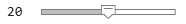# afterAll

Run function after all functions finish running in the background

## Syntax

``B = afterAll(A,fcn,n)``
``B = afterAll(A,fcn,n,PassFuture=true)``

## Description

example

````B = afterAll(A,fcn,n)` returns a `Future` object `B` and runs the function `fcn` automatically after all elements in the `Future` array `A` finish.MATLAB® runs the function `fcn` using the concatenated outputs from each element in `A`. The outputs `Y1,...,Ym` from each `Future` object are concatenated vertically, in linear index order. For example, if `A` is a two-element `Future` vector with outputs `y1` and `y2` from the first and second `Future` objects respectively, MATLAB runs `fcn([y1; y2])` after all elements in `A` finish.If the `Future` array `A` has `M` elements, MATLAB runs the function only once. When the scheduled function `fcn` finishes, the `Future` object `B` finishes.You create a `Future` object when: You run a function in the background using `backgroundPool`.You run a function on a parallel pool when you have Parallel Computing Toolbox™. For more information about using `afterAll` in parallel, see Use afterEach and afterAll to Run Callback Functions (Parallel Computing Toolbox).If any of the elements in `A` encounters an error, the `Error` property of `B` is an `MException`.```
````B = afterAll(A,fcn,n,PassFuture=true)` runs `fcn(A)` instead of using the concatenated outputs of each element in `A`.The `Error` property of `B` is an empty `MException` array, even if one or more elements in `A` encounter an error.```

## Examples

collapse all

This example shows how to use `afterEach` to update a wait bar with the progress of functions running in the background.

Create a wait bar, `w`.

`w = waitbar(0,'Please wait ...');`Set the number of iterations for your `for`-loop, `N`. Store the current number of completed iterations, `0`, and the total number of iterations, `N`, in the `UserData` property of the wait bar.

```N =20; w.UserData = [0 N];```

Run a `for`-loop with `N` iterations. In each iteration, use `parfeval` and `backgroundPool` to run `pause` in the background for a random number of seconds. Store each `Future` object in an array.

```for i = 1:N delay = rand; f(i) = parfeval(backgroundPool,@pause,0,delay); end```

Use the helper function `updateWaitbar` to update the waitbar after each `Future` finishes.

`afterEach(f,@(~)updateWaitbar(w),0);`

Use `delete` to close the wait bar after all the `Future` objects finish.

`afterAll(f,@(~)delete(w),0);`

Define Helper Function

Define the helper function u`pdateWaitbar`. The function increments the first element of the `UserData` property, then uses the vector to calculate the progress.

```function updateWaitbar(w) % Update a waitbar using the UserData property. % Check if the waitbar is a reference to a deleted object if isvalid(w) % Increment the number of completed iterations w.UserData(1) = w.UserData(1) + 1; % Calculate the progress progress = w.UserData(1) / w.UserData(2); % Update the waitbar waitbar(progress,w); end end```

## Input Arguments

collapse all

Input `Future` object, specified as a `parallel.Future` scalar or array.

MATLAB runs the function `fcn` after each element in `A` finishes. If any of the elements in `A` encounters an error and you specify `PassFuture` as `true`, `afterAll` still runs `fcn` using that element.

• If you specify `PassFuture` as `true`, the function is run as `fcn(Aj)` using each `Future` element `Aj` in `A`. If any of the elements in `A` encounters an error, `afterAll` does not finish with an error.

• Otherwise, the function is run as `fcn(X1,...,Xm)` using the outputs `X1,...,Xm` from each `Future` element in `A`. If any of the elements in `A` encounters an error, `afterAll` finishes with an error.

If the `Future` array has `M` elements, MATLAB runs the function `M` times. When the scheduled function `fcn` finishes, the `Future` object `B` finishes.

Example: ```A = parfeval(backgroundPool,@magic,1,3);```

Callback function to run, specified as a function handle.

Example: `fcn = @magic`

Number of output arguments, specified as a nonnegative integer scalar.

• If you specify `PassFuture` as `true`, `n` is the number of output arguments expected from running `fcn(A)` using the `Future` array `A`.

• Otherwise, `n` is the number of output arguments expected from running `fcn(Y1,...,Ym)` using the vertically concatenated outputs `Y1,...,Ym` from each element `Aj` in the `Future` array `A`.

Data Types: `single` | `double` | `int8` | `int16` | `int32` | `int64` | `uint8` | `uint16` | `uint32` | `uint64`

## Output Arguments

collapse all

Output `Future` object, returned as a `parallel.Future` object.

When you set `PassFuture`, you change the `Error` property of `B`:

• If `PassFuture` is `true`, the `Error` property of `B` is an empty `MException` array, even if one or more elements in `A` encounter an error.

• Otherwise, if any of the elements in `A` encounters an error, the `Error` property of `B` is an `MException` object.

To find the cause of the error, use the `cause` property of `B.Error`.